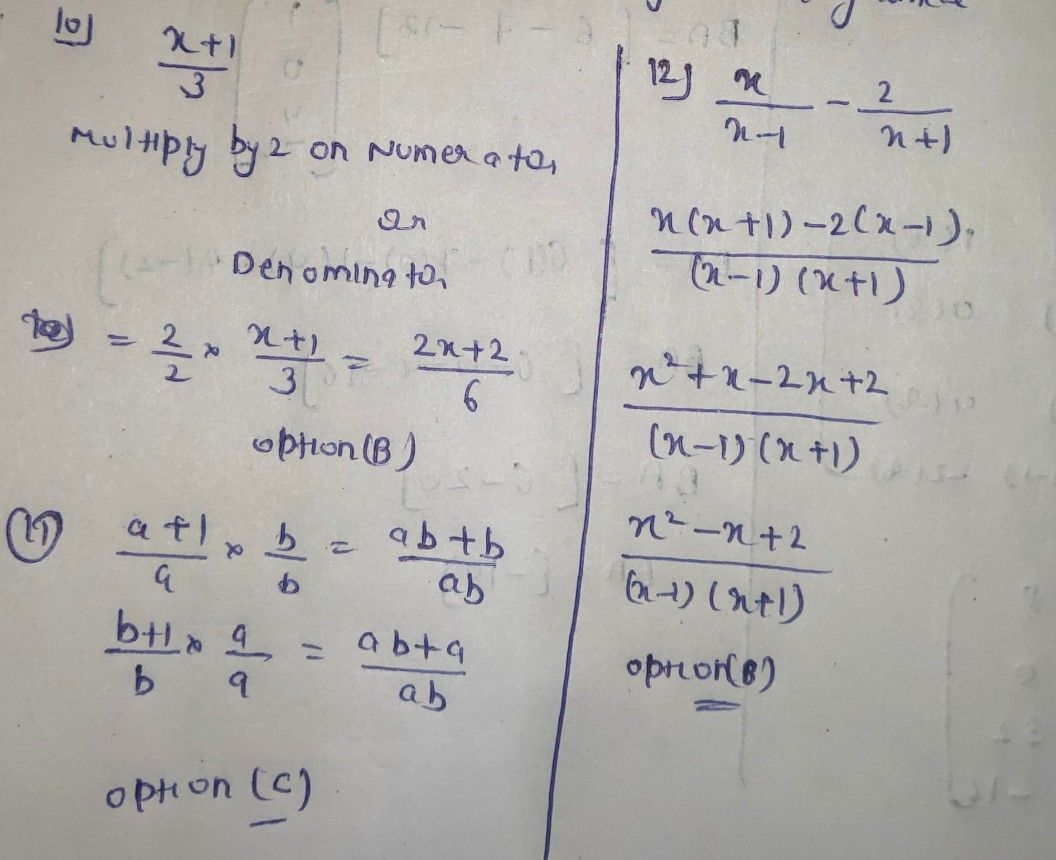Symbol
Problem10. Using the $lCD6$ look for the equivalent rational algebraic expression of $\dfrac {x+1} {3}$ 2x+1 B. $\dfrac {2x+2} {6}$ C. 6x+1 D. $\dfrac {6x+6} {3}$ 6. 11. Look for the equivalent rational aigebraic expression of each of a a+1 and b+1 b if the $LCDls$ ab. A. $\dfrac {ab+1} {ab}$ $\dfrac {ab+b} {ab}$ C. $\dfrac {ab+b} {ab}$ $\dfrac {ab+a} {ab}$ B. $\dfrac {ab-a} {ab}$ $\dfrac {ab-1} {ab}$ D. $\dfrac {ab-b} {ab}$ $\dfrac {ab-a} {ab}$ $ply^{\dfrac {x} {x-1}-\dfrac {2} {x+1}}$ 12. Write as one fraction and simplifyx-1 A. $\dfrac {x^{2}+x+2} {\left(x-1\right)\left(x+1\right)}$ C. $\dfrac {x^{2}-x-2} {\left(x-1\right)\left(x+1\right)}$ B. $\dfrac {x^{2}-x+2} {\left(x-1\right)\left(x+1\right)}$ D. $\dfrac {x^{2}+x-2} {\left(x-1\right)\left(x+1\right)}$
7th-9th grade
Algebra
Search count: 107
SolutionQanda teacher - AskhinaStudent
Thank you very muchQanda teacher - Askhina
welcome